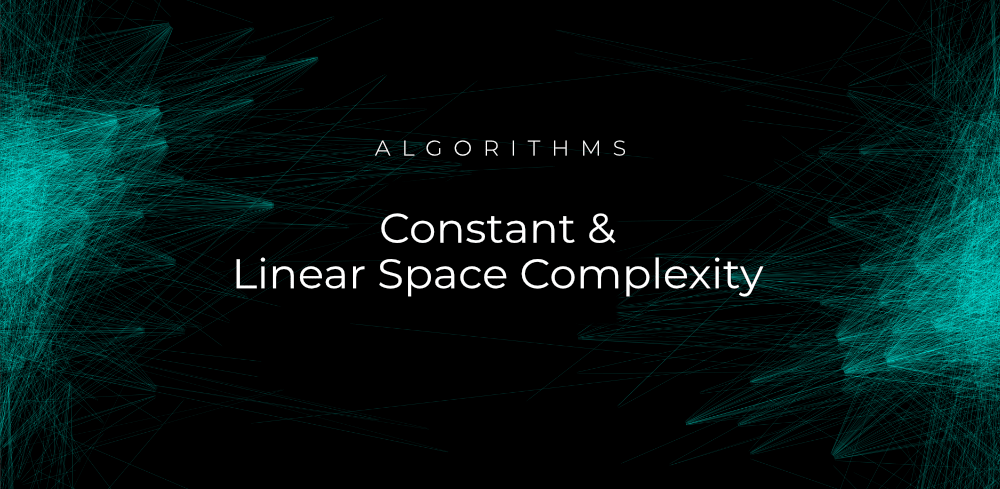# Constant & Linear Space Complexity in Algorithms

• Difficulty Level : Basic
• Last Updated : 22 Jun, 2021

Being a programmer, you might have solved a lot of programming challenges. In programming space and time complexity matters a lot when we need to execute a program. Our algorithm should be efficient, and it should take less time. Whenever you’re writing a solution to a program, some memory is required to complete, and it is important for our program to take less memory and execute within a given timeframe.For any program, memory may be used for the following…

1. Variables (This includes the constant values, temporary values)
2. Program Instruction
3. Execution

Space Complexity is the total amount of memory a program an algorithm takes to execute and produce the result. Many times programmers get confused about Auxiliary Space and Space Complexity. Both are different. In any algorithm, the extra space or the temporary space that we use is known as Auxiliary space.

`Space Complexity = Auxiliary Space + Input space`

In this article, we will understand the memory usage while execution, and we will understand the classification of space complexity. The algorithm uses memory space for three reasons…

1. Instruction Space: While writing an algorithm, the compiled version of instructions takes some amount of memory which is known as Instruction space.

2. Environmental Stack: It is required to store the environmental information needed to resume the suspended function. This is used when an algorithm is called inside another algorithm. In those situations, we push the current variables into the system stack, where we wait for further execution. After that, we call it inside the algorithm

Let’s take the example of two functions. Function X() and function Y(). Variables of function X() will be stored on the system stack temporarily, while function Y() is called and executed inside function X().

3. Data Space: During the execution of a program whatever space is required to store the constants and variable values are considered as Data Space.

Note: While writing an algorithm you only need to consider the Data Space. You don’t need to calculate the Instruction Space and Environmental Stack.

Now let’s understand the classification of space complexity. Space complexity can be calculated in two ways. It depends on the program. The program may be a constant program or a linear program.

### How to Calculate the Space Complexity?

In an algorithm, firstly you need to calculate the total amount of memory to evaluate the space complexity. You need to know the value of memory used by different types of data types of variables. For different operating systems or different machines. This value can be different for each machine, but the method remains the same.

Let’s take one of the examples for calculating space complexity…

```{
int x = a * b * c;
return(x);
}```

In the above expression, a, b, c, and x all are integer types. Each of them takes 4 bytes. Now we can calculate the total memory. This will be…

(4(4) + 4) = 20 bytes. Additional 4 bytes are for the return value. Here we need a fixed amount of memory for all the input. So this type of complexity is called Constant Space Complexity

Let’s move to another example…

```// n is the length of array a[]
int sum(int arr[], int n)
{
int sum = 0;  // 4 bytes for sum
for(int i = 0; i < n; i++) // 4 bytes for i
{
sum  = sum + arr[i];
}
return(sum);
}```
• In the above example, we need 4*n bytes of space for each element of the array.
• 4 bytes each for sum, n, i, and the return value.

So the total amount of memory will be (4n+12) which is increasing linearly with an increase in the input value n. This is called Linear Space Complexity. If you have a loop variable I, then the required space complexity will be 1 word.

If you have understood the concept of calculating the space complexity from the above example then following a similar approach you can calculate quadratic and other space complexity, as the complexity of an algorithm increases. Always try to minimize the space complexity of your algorithm. Lesser space makes your algorithm better and efficient.

### Summary

• O(1) Complexity: We consider constant space complexity when the program doesn’t contain any loop, recursive function, or call to any other functions.
• O(n) Complexity: We consider the linear space complexity when the program contains any loops.

### Space Complexity Cheat Sheet for Algorithms

• Bubble Sort: O(1)
• Selection Sort: O(1)
• Insertion Sort: O(1)
• Merge Sort: O(n)
• Quick Sort: O(n)
• Heap Sort: O(1)
• Radix Sort: O(n+k) where k — range of array elements.

### Final Thought

Space complexity plays a crucial role in determining the efficiency of an algorithm. Always try to implement an algorithm that takes less time. If a program takes a lot of memory space, the compiler will not let you run it. Always remember the below formula in space complexity

`Space Complexity = Auxiliary space + Space use by input values`
My Personal Notes arrow_drop_up